# High School Math : How to find the perimeter of a right triangle

## Example Questions

### Example Question #1 : How To Find The Perimeter Of A Right Triangle

What is the perimeter of a triangle with side lengths of 5, 12, and 13?

Possible Answers: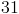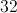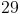Correct answer:Explanation:

To find the perimeter of a triangle you must add all of the side lengths together.

In this case our equation would look like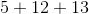Add the numbers together to get the answer.

### Example Question #2 : How To Find The Perimeter Of A Right Triangle

Three points in the xy-coordinate system form a triangle.

The points are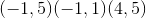.

What is the perimeter of the triangle?

Possible Answers: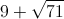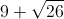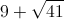Correct answer:Explanation:

Drawing points gives sides of a right triangle of 4, 5, and an unknown hypotenuse.

Using the pythagorean theorem we find that the hypotenuse is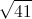.

### Example Question #3 : How To Find The Perimeter Of A Right Triangle

Find the perimeter of the following triangle: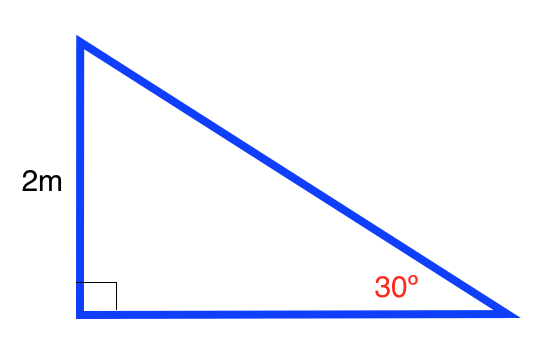Possible Answers: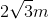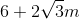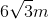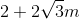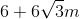Correct answer:Explanation:

The formula for the perimeter of a right triangle is: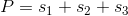whereis the length of a side.

Use the formulas for a a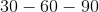triangle to find the length of the base. The formula for atriangle is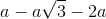.

Ourtriangle is: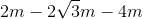Plugging in our values, we get: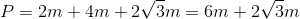### Example Question #4 : How To Find The Perimeter Of A Right Triangle

Find the perimeter of the following right triangle: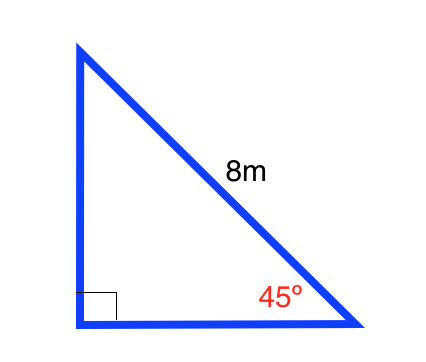Possible Answers: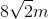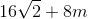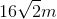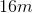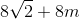Correct answer:Explanation:

The formula for the perimeter of a right triangle is:whereis the length of a side.

Use the formulas for a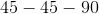triangle to find the length of the base and height. The formula for atriangle is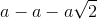Ourtriangle is: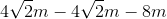Plugging in our values, we get: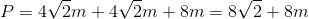### Example Question #5 : How To Find The Perimeter Of A Right Triangle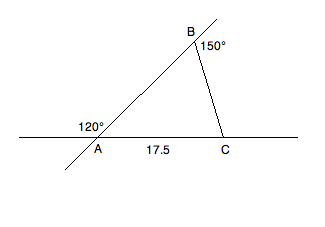Based on the information given above, what is the perimeter of triangle ABC?

Possible Answers: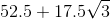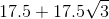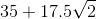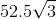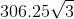Correct answer:Explanation: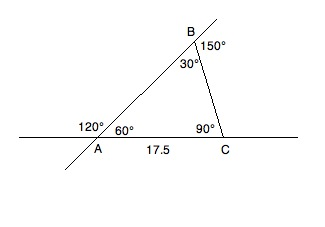Consult the diagram above while reading the solution. Because of what we know about supplementary angles, we can fill in the inner values of the triangle. Angles A and B can be found by the following reductions:

A + 120 = 180; A = 60

B + 150 = 180; B = 30

Since we know A + B + C = 180 and have the values of A and B, we know:

60 + 30 + C = 180; C = 90

This gives us a 30:60:90 triangle. Now, since 17.5 is across from the 30° angle, we know that the other two sides will have to be √3 and 2 times 17.5; therefore, our perimeter will be as follows: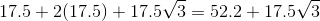### All High School Math Resources